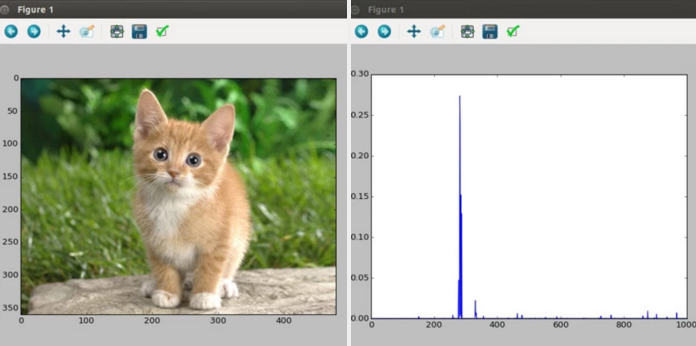## 一點點幫助入門的細節

$./scripts/download_model_binary.py ./models/bvlc_googlenet 上面這個指令會將GoogLeNet的model下載到caffe/models/bvlc_googlenet，假設已經下載好model，接下來就可以用一個小程式來跑跑看GoogLeNet了: import numpy as np import matplotlib.pyplot as plt # Make sure that caffe is on the python path: caffe_root = '../' # this file is expected to be in {caffe_root}/examples import sys sys.path.insert(0, caffe_root + 'python') sys.path.append(&quot;/usr/lib/python2.7/dist-packages/&quot;) import caffe # Set the right path to your model definition file, pretrained model weights, # and the image you would like to classify. MODEL_FILE = '../models/bvlc_googlenet/deploy.prototxt' PRETRAINED = '../models/bvlc_googlenet/bvlc_googlenet.caffemodel' IMAGE_FILE = 'images/cat.jpg' caffe.set_mode_cpu() net = caffe.Classifier(MODEL_FILE, PRETRAINED, mean=np.load(caffe_root + 'python/caffe/imagenet/ilsvrc_2012_mean.npy').mean(1).mean(1), channel_swap=(2,1,0), raw_scale=255, image_dims=(256, 256)) input_image = caffe.io.load_image(IMAGE_FILE) plt.imshow(input_image) plt.show() prediction = net.predict([input_image]) plt.plot(prediction) plt.show() print 'predicted class:', prediction.argmax()  接下來只要執行(因為程式放在examples資料夾底下): $python ./examples/googlenet_example.py## 串接ros_caffe

@pojenlai 演算法工程師，對機器人跟電腦視覺有少許研究，最近在鍛鍊自己的執行力